# NCERT Solution (Ex 1.1) - Chapter 1: Rational Number, Maths, Class 8 Notes - Class 8

## Class 8: NCERT Solution (Ex 1.1) - Chapter 1: Rational Number, Maths, Class 8 Notes - Class 8

The document NCERT Solution (Ex 1.1) - Chapter 1: Rational Number, Maths, Class 8 Notes - Class 8 is a part of Class 8 category.
All you need of Class 8 at this link: Class 8

Exercise 1.1 (Rational Number)

Question 1:
Using appropriate properties find: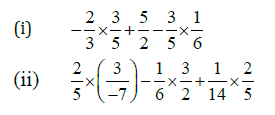(i)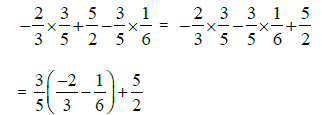[Using associative property]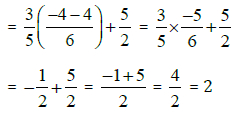[Using associative property]

(II)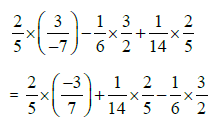[Using associative property]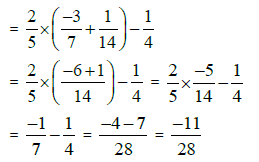[Using distributive property]

Question 2:
Write the additive inverse of each of the following:
(i) 2/8
(ii) -5/9
(iii) -6/-5
(iv) 2/-9
(v) 19/-6

We know that additive inverse of a rational number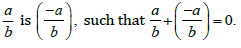(i) Additive inverse of 2/8 is -2/8
(ii) Additive inverse of -5/9 is 5/9
(iii) Additive inverse of  -6/-5 is -6/5
(iv) Additive inverse of 2/-9 is 2/9
(v) Additive inverse of19/-6  is 19/6

Question 3:
Verify that -(-x)= x for:
(i)x= 11/15
(ii)x= 13/17

(i) Putting x= 11/15  in  -(-x) =x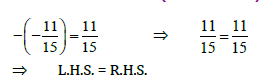Hence, verified.

(ii)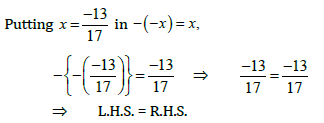Hence, verified.

Question 4:
Find the multiplicative inverse of the following: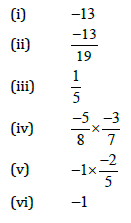We know that multiplicative inverse of a rational number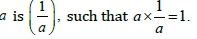(i) Multiplicative inverse of  - 13 is -1/13

(ii) Multiplicative inverse of -13/19 is -19/13

(iii) Multiplicative inverse of 1/5 is 5

(iv) Multiplicative inverse of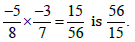(v) Multiplicative inverse of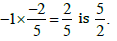(vi) Multiplicative inverse of -1 is 1/-1

Question 5:
Name the property under multiplication used in each of the following: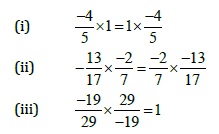(i) 1 is the multiplicative identity.
(ii) Commutative property.
(iii) Multiplicative Inverse property.

Question 6:
Multiply 6/13 by the reciprocal of -7/
16
The reciprocal of -7/16 is -16/7
According to the question,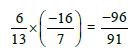Question 7:
Tell what property allows you to compute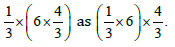By using associative property of multiplication, a x (b x c) = (a x b) x c.

Question 8:
Is 8/9 the multiplicative inverse of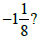Why or why not?

Since multiplicative inverse of a rational number a is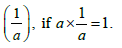Therefore,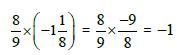But its product must be positive 1.
Therefore, 8/9 is not the multiplicative inverse of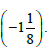Question 9:
Is 0.3 the multiplicative inverse of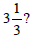Why or why not?

Since multiplicative inverse of a rational number a isTherefore,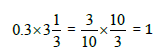Therefore, Yes 0.3 is the multiplicative inverse of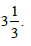Question 10:
Write:
(i) The rational number that does not have a reciprocal.
(ii) The rational numbers that are equal to their reciprocals.
(iii) The rational number that is equal to its negative.

(i) 0
(ii) 1 and -1
(iii) 0

The document NCERT Solution (Ex 1.1) - Chapter 1: Rational Number, Maths, Class 8 Notes - Class 8 is a part of Class 8 category.
All you need of Class 8 at this link: Class 8Use Code STAYHOME200 and get INR 200 additional OFF

Track your progress, build streaks, highlight & save important lessons and more!

,

,

,

,

,

,

,

,

,

,

,

,

,

,

,

,

,

,

,

,

,

,

,

,

,

,

,

;